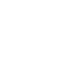Menu# Maximum weight, length and girthWeight up to 31.5 kg

The maximum permitted weight is 31.5 kg (for each individual shipment)Length up to 175 cm

The maximum length of the longest side is 175 cm.Girth 300 cm

The maximum girth 300 cm (2x width + 2x height + 1x length).Weight up to 31,5 kg

The maximum permitted weight is 31,5 kg (for each individual shipment).Lenght up to 175 cm

The maximum lenght of the longest side 175 cm.Girth 300 cm

The maximum girth 300 cm (2x width + 2x height + 1x lenght).Weight up to 15 kg

The maximum permitted weight is 15 kg (for each individual shipment).Length up to 100 cm

Maximum length of the longest side 100 cm.Girth 250 cm

Maximum girth 250 cm (2x width + 2x height + 1x length).Weight up to 15 kg

The maximum permitted weight is 15 kg.Maximum dimensions

Max. dimensions for parcels sent via boxes are 40 x 75 x 60 (W x H x D; in cm).

# Volumetric weight

Airfreight parcels are priced on the basis of volumetric weight in cases where this exceeds the actual weight in kg. The volumetric weight indicates how much space a parcel occupies within the aircraft in relation to its weight and, in the interests of economic and ecological loading of passenger aircraft, is calculated using the following formula:

Length (cm) x width (cm) x height (cm) / 5,000 (cm3/KG)

The volumetric weight is only relevant to DPD EXPRESS international parcels.

# Girth and Volumetric Weight Calculator

Normal Girth Calculator
Parcelshop Calculator

Height (cm)Width (cm)Length (cm)

The combined dimensions of your parcel are:

cm

The volumetric weigth of your parcel:

KG

NOTE: This is a computed value which was not measured with calibrated scales - please see the note in the first paragraph.